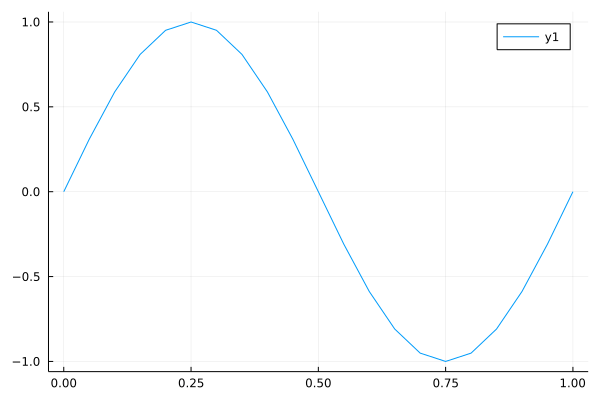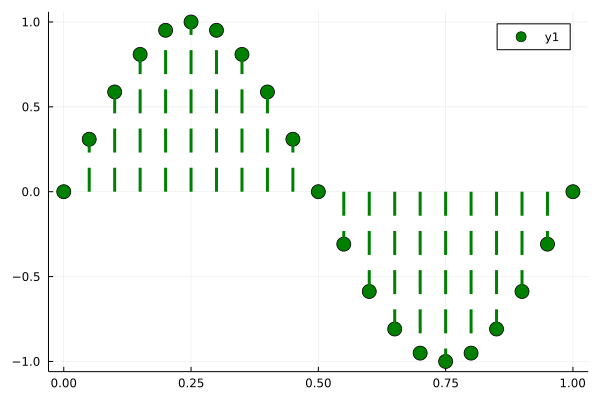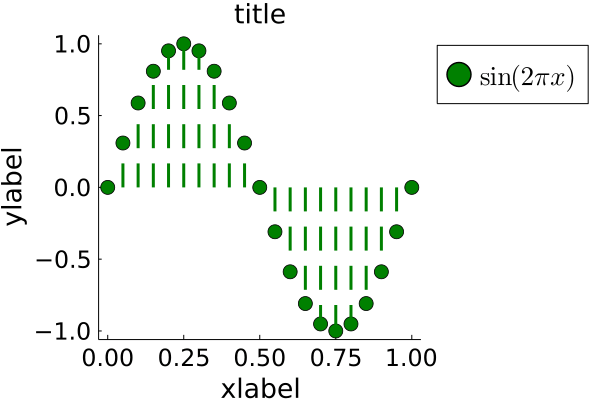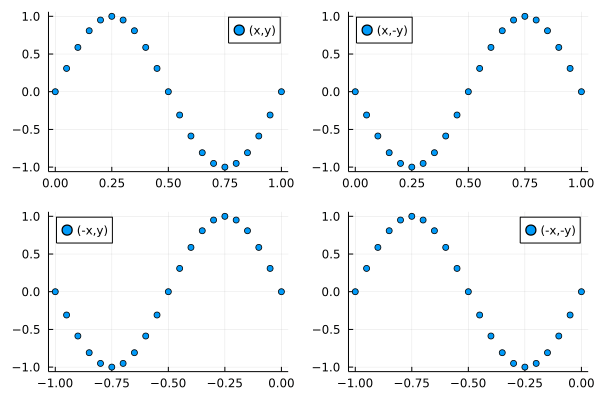There are many visualization libraries to choose from in the Julia ecosystem (see below on this page). Arguably, the Plots.jl library is the standard plotting tool in the Julia ecosystem. It in turn provides a single API to access multiple “backends”, which include:

Backend Documentation
Matplotlib (Pyplot) Pyplot
Plotly Plotly
GR GR

To select one of these different backends, we simply call the corresponding command. For example, to load Plots.jl and use the GR backend (which is the default), we would do:

using Plots
gr()


You can check the official documentation of Plots.jl here.

While the Tutorial section of the documentation can help you get started, I found it a little overwheliming at first sight. Over time, I ended up compiling a list of the properties I most frequently use, which I share in this page.

## Simple Plots with Plots.jl and GR

The simplest kind of plots are line plots, which can be produced given at least two vectors x and y.

using Plots
gr()

x = 0:0.05:1;
y = sin.(2π*x);
plot(x,y)## Styling Lines and Markers

To begin styling our plot, we can begin with setting the following Series attributes:

Series attributes Type
seriestype :path, :sticks, :scatter, :bar
lw Float
seriescolor Color Type
marker :d, :hex,
markersize Float
markerstrokecolor Color Type

which will allow us to style the line and optionally add different kinds of markers on top.

As for the colors, Color Type can stand for any symbol of the following list, like :darkblue, :firebrick, etc.

Note that changing the seriestype of a column is equivalent to using commands such as scatter(x,y), bar(x,y), etc.

Let’s see an example

plot(   x, y,
seriestype=:sticks,
linestyle=:dash,
lw = 3,
seriescolor = :green,
marker = :circle,
markersize = 8,
markercolor = :green,
markerstrokecolor = :green,
)More succintly, we can also use the equivalent syntax:

plot(   x, y,
line=(3,:green,:dash,:sticks),
marker=(:circle,8,:green,:green)
)


Note that, remarkably, the line or market tuples can contain their elements in different orders, so line=(:path,:dash,:green,2) or line=(2,:green,:dash,:path) will work as well.

## Grid Lines

There are a few attributes related to the grid lines.

Series attributes Type
grid boolean
gridlinewidth Number
gridalpha Number in [0,1]
gridstyle :solid, :dash, :dot, :dashdot, :dashdotdot

In particular, grid=false will turn the grid lines off entirely.

## Equal Aspect Ratio

In order to get equally scaled axes with Plots.jl, we set the attribute aspect_ratio = :equal. This is the Julia equivalent to Matlab’s “axis equal” for example.

## Text and Font Sizes

There are a number of typical places where we might want to include text within a figure. The title, labels for the x and y axes, or the legend for the line plots. Some of the most useful Subplot Attributes to do this are:

Subplot Attribute Type
title, xlabel, ylabel, label string
titlefontsize, guidefontsize, tickfontsize, legendfontsize Int
legend :right, :left, :top, :bottom, etc

Some other useful values for the legend position are: :topright, :topleft, :bottomright, :bottomleft, :outerright, :outerleft, :outertopright, :outertopleft, :outerbottomright, :outerbottomleft.

An other very useful package that can lead to fantastic quality plots is LaTeXStrings (mind the uppercases).

When we are using this package, simply preceding a string with an L, it will tell Plots.jl that we want to render it in Latex (e.g. L"\sin(2\pi x)")

Let’s see how all this fits together:

using Plots
using LaTeXStrings

plot(   x, y,
line=(3,:green,:dash,:sticks),
marker=(:circle,8,:green,:green),
title="title",
xlabel="xlabel",
ylabel="ylabel",
label=L"\sin(2\pi x)",
legend=:outertopright,
titlefontsize=18,
guidefontsize=18,
tickfontsize=16,
legendfontsize=18,
grid=false
)## Subplots and Layouts

To compile multiple plots into a single figure, we need to adjust the layout.

The simplest way to do this is to create the plots sequentially, as follows:

using Plots
p1 = scatter(x, y, label="(x,y)")
p2 = scatter(x, -y, label="(x,-y)", legend=:topleft)
p3 = scatter(-x, y, label="(-x,y)", legend=:topleft)
p4 = scatter(-x, -y, label="(-x,-y)")

plot(p1,p2,p3,p4, layout = (2,2) )## Other Visualization Packages

There are multiple plotting packages for Julia worth checking:

Package Description Examples Tutorial
StatsPlots.jl A drop-in replacement for Plots.jl that contains specialized statistical plotting functionalities. StatsPlots.jl repository Plots.jl docs
Makie.jl A high-performance plotting ecosystem with OpenGL, Cairo and WebGL backends. It’s great for publication-quality plotting, but can be a little bit slow to load and use Docs
VegaLite A Julia wrapper for the Vega-Lite library. Great for interactive graphics. Docs.
Gadfly Based on the R package gglot2, very well suited for statistics and machine learning. Docs

Detailed documentation can be found in each package, and in the referenced tutorials and examples pages.